# PSAT Math : How to express a fraction as a ratio

## Example Questions

### Example Question #1 : How To Express A Fraction As A Ratio

express 7/8 as a ratio

8:7

not possible to express as a ratio

7:8

0.875

1.15

7:8

Explanation:

a ratio that comes from a fraction is the numerator: denominator

7/8 = 7:8

### Example Question #2 : How To Express A Fraction As A Ratio

1 meter contains 100 centimeters.

Find the ratio of 1 meter and 40 centimeters to 1 meter:

12:5

7:5

3:2

2:3

9:6

7:5

Explanation:

1m 40cm = 140cm. 1m = 100cm. So the ratio is 140cm:100cm. This can be put as a fraction 140/100 and then reduced to 14/10 and further to 7/5. This, in turn, can be rewritten as a ratio as 7:5.

### Example Question #1 : How To Express A Fraction As A Ratio

When television remotes are shipped from a certain factory, 1 out of every 200 is defective. What is the ratio of defective to nondefective remotes?

200:1

1:200

1:199

199:1

1:199

Explanation:

One remote is defective for every 199 non-defective remotes.

### Example Question #2 : How To Express A Fraction As A Ratio

On a desk, there arepapers for everypaper clips andpapers for everygreeting card. What is the ratio of paper clips to total items on the desk?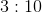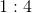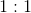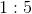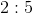Explanation:

Begin by making your life easier: presume that there arepapers on the desk. Immediately, we know that there arepaper clips. Now, if there arepapers, you know that there also must begreeting cards. Technically you figure this out by using the ratio: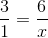By cross-multiplying you get: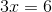Solving for, you clearly get.

(Many students will likely see this fact without doing the algebra, however. The numbers are rather simple.)

Now, this means that our desk has on it:paperspaper clipsgreeting cards

Therefore, you havetotal items.  Based on this, your ratio of paper clips to total items is: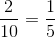, which is the same as.

### Example Question #3 : How To Express A Fraction As A Ratio

In a classroom ofstudents, each student takes a language class (and only one—nobody studies two languages).take Latin,take Greek,take Anglo-Saxon, and the rest take Old Norse. What is the ratio of students taking Old Norse to students taking Greek?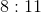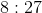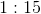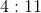Explanation:

To begin, you need to calculate how many students are taking Old Norse. This is: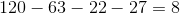Now, the ratio of students taking Old Norse to students taking Greek is the same thing as the fraction of students taking Old Norse to students taking Greek, or: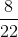Next, just reduce this fraction to its lowest terms by dividing the numerator and denominator by their common factor of: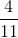This is the same as.

### Example Question #4 : How To Express A Fraction As A Ratio

In a garden, there arepansies,lilies,roses, andpetunias. What is the ratio of petunias to the total number of flowers in the garden?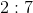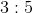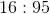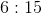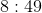Explanation:

To begin, you need to do a simple addition to find the total number of flowers in the garden: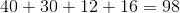Now, the ratio of petunias to the total number of flowers in the garden can be represented by a simple division of the number of petunias by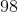. This is: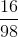Next, reduce the fraction by dividing out the commonfrom the numerator and the denominator: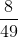This is the same as.